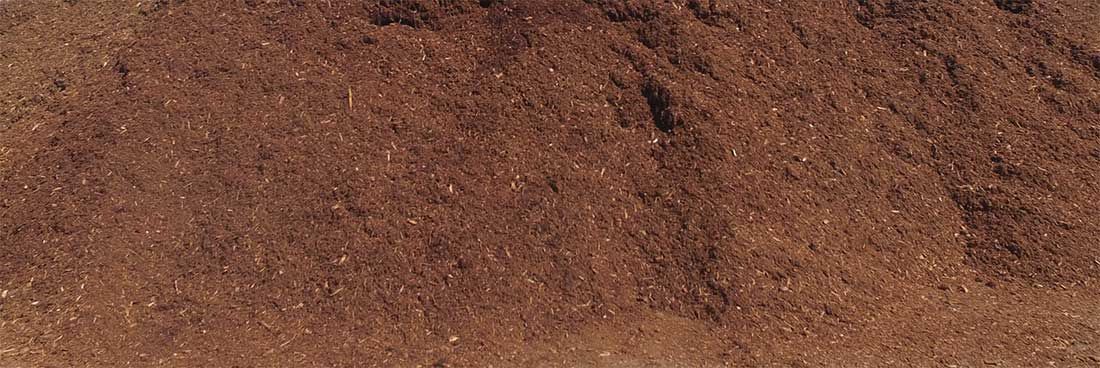Mulch Coverage CalculatorHow Much Mulch Do I Need?

To ease the stress of deciding how many yards of mulch you'll need, use our mulch calculator to find the correct amount for your project.

Enter the approximate square footage of area you want to cover, then the desired depth (standard depth for mulch is 3"), then click Calculate. The calculator will tell you approximately how many cubic yards of mulch you'll need (based on typical coverage of 100 sq. ft./cubic yard, 3" deep).

Then call the S & J Exco, Inc at (508) 398-4000. We'll be happy to help you make sure you get the right product, and the right amount!

Mulch Coverage Calculator

Area: sq. ft.

Depth: inches

You will require cubic yards of mulch